# A tumbling T-handle in space: the Dzhanibekov effect

The video in Figure 1 of a tumbling T-handle on the International Space Station is a wonderful illustration of the instability of rotation about an asymmetric object’s intermediate principal axis. This instability is also known as the Dzhanibekov effect after the Soviet cosmonaut Vladimir Dzhanibekov who discovered this effect on the MIR space station in 1985. The T-handle’s rotational motion is well approximated by considering the object to be rigid and modeling its rotational behavior via a balance of angular momentum.

Contents

## Equations of motion

Suppose we parameterize the rotation of the T-handle by a 3-1-3 set of Euler angles: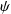,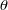, and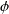. As illustrated in Figure 2, this sequence of rotations relates the space-fixed basis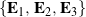to the corotational basis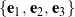associated with the T-handle’s principal axes, with the intermediate axis aligned with the object’s longitudinal axis.

The angular momentum of the T-handle about its mass center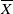is given by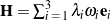, whereare the principal moments of inertia (which are distinct for an asymmetric body) and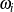are the corotational components of angular velocity: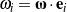. Neglecting the small resultant moment acting on the T-handle due to the central force field while orbiting the earth, a balance of angular momentum with respect to the mass center yields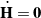, resulting in the equations of motion

(1)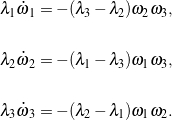For a 3-1-3 set of Euler angles,are related to the Euler angles,, andand their rates of change as follows:

(2)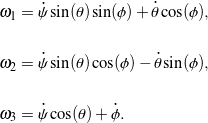Sets (1) and (2) constitute a system of first-order differential equations that solve for the T-handle’s orientation over time. These equations may be conveniently expressed in the formfor numerical integration in MATLAB, where we take the state vector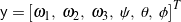:

(3)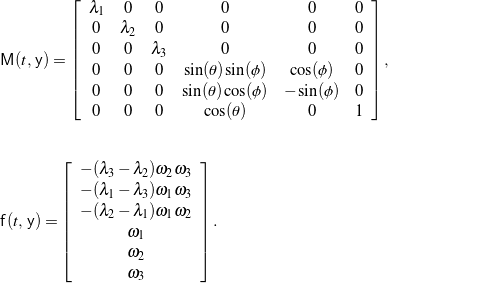## Simulation and animation

To demonstrate instability of rotation about the intermediate principal axis, suppose the T-handle is initially spinning about this axis at a rate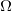with small perturbationsin the initial angular velocity about the other two principal axes: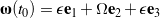. For convenience, let the T-handle’s initial orientation be defined as,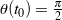rad (to avoid the singularity in the 3-1-3 set of Euler angles), and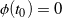. The resulting motion of the T-handle, which is very similar to the observed behavior in Figure 1, is animated in Figure 3 for a particular choice of physical and simulation parameters. It can also be shown that the simulated motion conserves both energy and angular momentum: the energy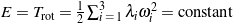, and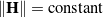.